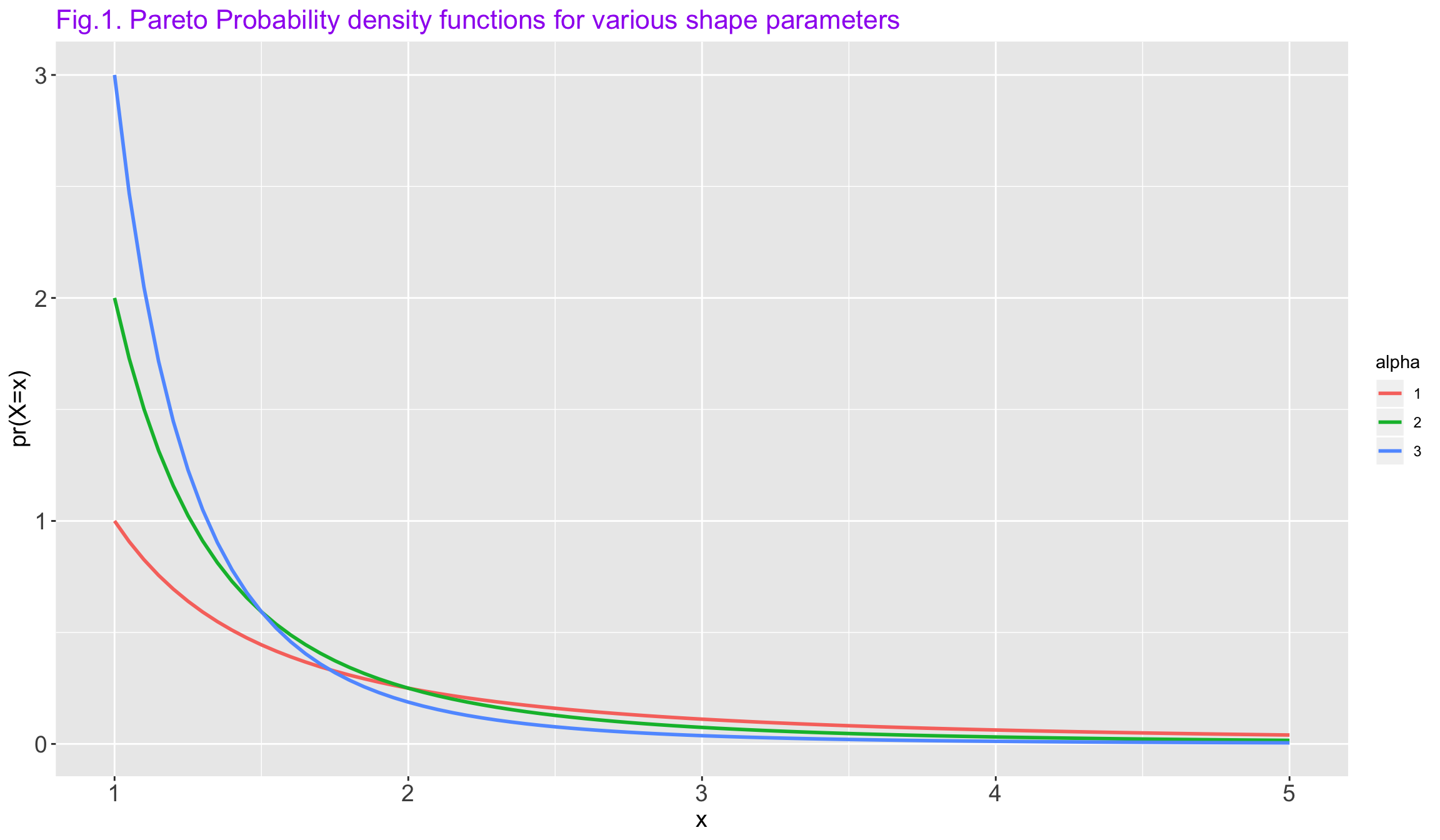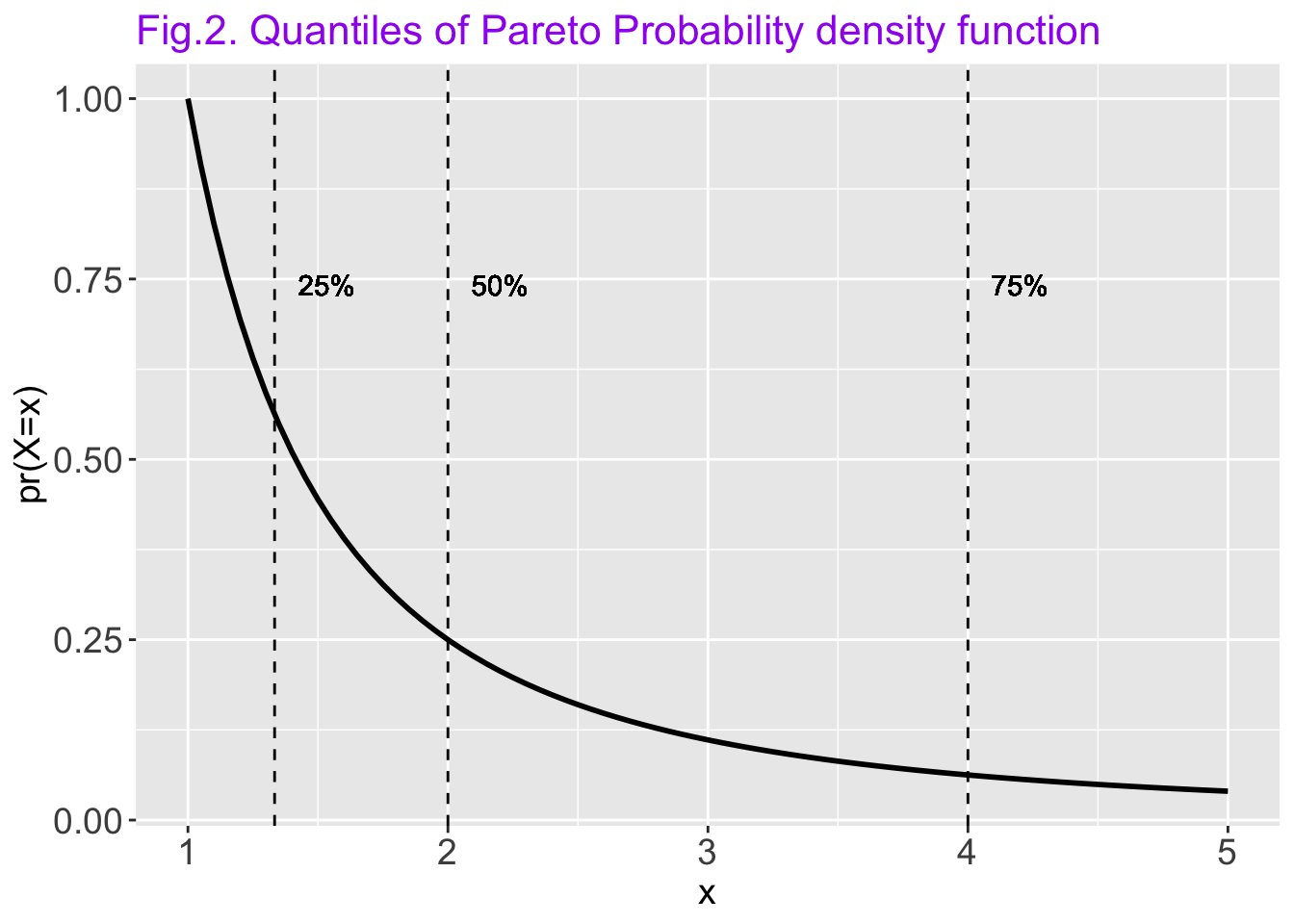## Home

The Pareto distribution is a power-law probability distribution that is used in description of social, scientific, geophysical, actuarial, and many other types of observable phenomena. Originally applied to describing the distribution of wealth in a society, fitting the trend that a large portion of wealth is held by a small fraction of the population, the Pareto distribution has colloquially become known and referred to as the Pareto principle, or “80-20 rule”. This rule states that, for example, 80% of the wealth of a society is held by 20% of its population. However, the Pareto distribution only produces this result for a particular power value, $$\alpha$$ ($$\alpha$$= log45 ≈ 1.16). While $$\alpha$$ is variable, empirical observation has found the 80-20 distribution to fit a wide range of cases, including natural phenomena and human activities (Wikipedia).

The probability density function of the common pareto distibution is:

$$f(x) = (x_{m}/x)^\alpha$$

where $$x_{m}$$ is the (necessarily positive) minimum possible value of X. It is scale parameter, and α is a positive parameter. It is a shape parameter.

x = seq(1, 5,0.05)
a3 = 3/x^(4)
a2 = 2/x^(3)
a1 = 1/x^(2)
df = bind_rows(data_frame(x = x, p=a3, alpha='3'),data_frame(x = x, p=a2, alpha='2'),data_frame(x = x, p=a1, alpha='1'))
df %>% ggplot(aes(x = x, y = p, group = alpha)) + geom_line(aes(color = alpha), size =1) + xlim(1, 5) +
ggtitle('Fig.1. Pareto Probability density functions for various shape parameters ') + ylab('pr(X=x)')+
theme(axis.title = element_text(size=14), plot.title = element_text(size = 16,colour="purple"),
axis.text = element_text(size=14))The Pareto distribution has a very long right-hand tail. It is often applied in the study of socioeconomic data, including the distribution of income, firm size, population, and stock price fluctuations.The Pareto distribution takes values on the positive real line. All values must be larger than the “location” parameter η, which is really a threshold parameter. There are three kinds of Pareto distributions. The one described here is the Pareto distribution of the first kind.

The Pareto distribution is related to the exponential distribution and logistic distribution as follows. Let X denote a Pareto random variable with location=η and shape=θ. Then log(X/η) has an exponential distribution with parameter rate=θ, and -log{ [(X/η)^θ] - 1 } has a logistic distribution with parameters location=0 and scale=1.

x = seq(1, 5,0.05)
a = (1/x)^(2)

quantiles = qpareto(c(0.25,0.5,0.75), location = 1, shape = 1)

df = data_frame(x = x, p=a)
df %>% ggplot(aes(x = x, y = p)) + geom_line(size = 1) +
ggtitle('Fig.2. Quantiles of Pareto Probability density function ') + ylab('pr(X=x)')+
theme(axis.title = element_text(size=14), plot.title = element_text(size = 16,colour="purple"),
axis.text = element_text(size=14)) + geom_vline(xintercept = quantiles, linetype = "dashed") + geom_vline(xintercept = quantiles, linetype = "dashed") + geom_vline(xintercept = quantiles, linetype = "dashed") + geom_text(aes(quantiles+0.2,0.7, label = '75%', vjust = -1)) +
geom_text(aes(quantiles+0.2,0.7, label = '50%', vjust = -1)) + geom_text(aes(quantiles+0.2,0.7, label = '25%', vjust = -1))We can estimate the parameters of data from Pareto distribution using maximum-likelihood or least-squares estimation.

#### Generate random numbers with pareto distribution

x = rpareto(100, location = 10, shape = 15)  

#### Estimate the parameters of the pareto distribution generated above.

estimated = eqpareto(x)
estimated

Results of Distribution Parameter Estimation
--------------------------------------------

Assumed Distribution:            Pareto

Estimated Parameter(s):          location = 10.00388
shape    = 15.05886

Estimation Method:               mle

Estimated Quantile(s):           Median = 10.47511

Quantile Estimation Method:      Quantile(s) Based on
mle Estimators

Data:                            x

Sample Size:                     100

#### calculate quantiles

qpareto(c(0.25,0.5,0.75, 0.9, 0.95), location = estimated$parameters, shape = estimated$parameters)
 10.19683 10.47511 10.96854 11.65667 12.20575

#### Check Visually

sort(x)
   10.00388 10.00706 10.01199 10.01258 10.01411 10.03796 10.04452
 10.05503 10.06279 10.06333 10.06843 10.06961 10.08429 10.09562
 10.09610 10.10844 10.11032 10.12467 10.12725 10.14430 10.15503
 10.15628 10.16288 10.16458 10.16846 10.17358 10.17831 10.17968
 10.22157 10.23495 10.23575 10.24469 10.24570 10.24872 10.26994
 10.30216 10.31218 10.33517 10.33581 10.35012 10.36778 10.37075
 10.37726 10.37846 10.37946 10.38815 10.39719 10.40092 10.40218
 10.40851 10.40871 10.41519 10.41674 10.42427 10.43639 10.44916
 10.45634 10.45729 10.46422 10.48859 10.49271 10.53899 10.58029
 10.58335 10.60893 10.61784 10.62240 10.64732 10.65105 10.65319
 10.67771 10.68119 10.68276 10.79123 10.79805 10.85948 10.87706
 10.89473 10.89521 10.94631 10.99511 11.01007 11.02911 11.17377
 11.24805 11.31271 11.38090 11.65450 11.74115 11.81652 11.81735
 11.86808 11.93101 13.10080 13.50952 13.52974 13.55708 13.59206
 13.88110 13.95104

x = rpareto(100000, location = 10, shape = 15)
estimated = eqpareto(x)
simulated2 = rpareto(estimated$sample.size, location = estimated$parameters, shape = estimated\$parameters)
d = bind_rows(data_frame(data = 'simulated1', value = x), data_frame(data = 'simulated2', value = simulated2))
d %>% ggplot(aes(value, fill = data, color = data)) + geom_density(alpha = 0.1)